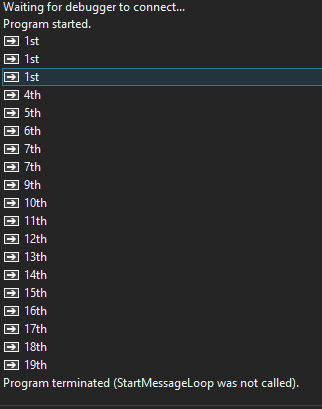# Android Code SnippetStudent Position Ranking

Hello everyone and Happy Holidays
So I was searching the community for a snippet for student ranking for a system I was building last month but never came accross a what I needed. I found a JavaScript on stackoverflow and re-wrote it in b4x
So I'm sharing for future/existing members who may need it.

Original from StackOverflow:
``````function calculatePosition(data){
var json = data;
json.sort((a, b) => b.total - a.total);
for (let i = 0; i < json.length; i++) {
let totalPoints = json[i].total;
let studentsWithRank = json.filter(student => student.total === totalPoints);
for (let student of studentsWithRank) {
student.rank = i + 1;
}
i += studentsWithRank.length - 1;
}
console.log(json);
return json;
}``````

The B4X Code Below

The Type can be changed to anything as long as there is something to enable ranking (ie. TotalScore, Rank)
Data Type:
``Type StudentScore(Total As Int, Year As String, StudentID As String, Term As Int, Class As String, Rank As Int)``

Filters the List to find similar scores and returns a list(rList).
Filter - is the item to be filtered out in the List
Filter Array or List:
``````Public Sub FilterList(List As List, Filter As Object) As List
Dim rList As List : rList.Initialize
For i = 0 To List.Size-1
Dim score As StudentScore = List.Get(i)
If score.Total == Filter Then
End If
Next
Return rList
End Sub``````

This performs the overall calculations returning a List with the Ranks assigned
Calculate Position:
``````Public Sub CalculatePosition(List As List) As List
Dim posList As List : posList.Initialize
For i = 0 To List.Size-1
Dim score As StudentScore = List.Get(i)
Dim totalPoints As Int = score.Total
Dim studentsWithRank As List = FilterList(List,totalPoints)
For Each student As StudentScore In studentsWithRank
student.Rank = i + 1
Log(student.Rank)
Next
i = i + studentsWithRank.Size-1
Next
Return posList
End Sub``````

Similar to CalculatePosition but only gets one Students position
Get Position:
``````Public Sub GetPosition(List As List, StudentID As String) As Int
Dim Position As Int
Dim posList As List : posList.Initialize
For i = 0 To List.Size-1
Dim score As StudentScore = List.Get(i)
Dim totalPoints As Int = score.Total
Dim studentsWithRank As List = FilterList(List,totalPoints)
For Each student As StudentScore In studentsWithRank
student.Rank = i + 1
Next
i = i + studentsWithRank.Size-1
Next
For i = 0 To posList.Size-1
Dim score As StudentScore = posList.Get(i)
If score.StudentID == StudentID Then
Position = score.Rank
End If
Next
Return Position
End Sub``````

Attached the number suffix(ie 1st, 2nd, 3rd)
Ordinal Suffix Of:
``````public Sub OrdinalSuffixOf(i As Int) As String
If i == 0 Then
Return i
Else
Dim j As Int = i Mod 10
Dim k As Int = i Mod 100
If (j == 1 And k <> 11) Then
Return i & "st"
End If
If (j == 1 And k <> 11) Then
Return i & "st"
End If
If (j == 2 And k <> 12) Then
Return i & "nd"
End If
If (j == 3 And k <> 13) Then
Return i & "rd"
End If
Return i & "th"
End If
End Sub``````

Data to be based can be JSON, an Array or List with objects
Usage Sample:
``````    Dim List As List : List.initialize
Dim DataString As String = \$"[{"total":603,"yeargroup":"2022\/2023","student_id":70,"term":3,"class":"KG 1"},{"total":603,"yeargroup":"2022\/2023","student_id":74,"term":3,"class":"KG 1"},{"total":603,"yeargroup":"2022\/2023","student_id":124,"term":3,"class":"KG 1"},{"total":168,"yeargroup":"2022\/2023","student_id":82,"term":3,"class":"KG 1"},{"total":166,"yeargroup":"2022\/2023","student_id":72,"term":3,"class":"KG 1"},{"total":163,"yeargroup":"2022\/2023","student_id":81,"term":3,"class":"KG 1"},{"total":153,"yeargroup":"2022\/2023","student_id":472,"term":3,"class":"KG 1"},{"total":153,"yeargroup":"2022\/2023","student_id":84,"term":3,"class":"KG 1"},{"total":150,"yeargroup":"2022\/2023","student_id":77,"term":3,"class":"KG 1"},{"total":148,"yeargroup":"2022\/2023","student_id":79,"term":3,"class":"KG 1"},{"total":142,"yeargroup":"2022\/2023","student_id":76,"term":3,"class":"KG 1"},{"total":138,"yeargroup":"2022\/2023","student_id":73,"term":3,"class":"KG 1"},{"total":136,"yeargroup":"2022\/2023","student_id":471,"term":3,"class":"KG 1"},{"total":135,"yeargroup":"2022\/2023","student_id":75,"term":3,"class":"KG 1"},{"total":131,"yeargroup":"2022\/2023","student_id":83,"term":3,"class":"KG 1"},{"total":125,"yeargroup":"2022\/2023","student_id":80,"term":3,"class":"KG 1"},{"total":121,"yeargroup":"2022\/2023","student_id":473,"term":3,"class":"KG 1"},{"total":102,"yeargroup":"2022\/2023","student_id":71,"term":3,"class":"KG 1"},{"total":98,"yeargroup":"2022\/2023","student_id":470,"term":3,"class":"KG 1"}]"\$
Dim DataList As List
Dim Parser As JSONParser
Parser.Initialize(DataString)
DataList = Parser.NextArray
For s = 0 To DataList.Size-1
Dim m As Map = DataList.Get(s)
Dim score As StudentScore
score.Initialize
score.Total = m.Get("total")
score.Year = m.Get("yeargroup")
score.StudentID = m.Get("student_id")
score.Term = m.Get("term")
score.class = m.Get("class")
Log(OrdinalSuffixOf(GetPosition(List,score.StudentID)))
Next``````

##### Member•